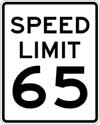#Difference Between Similar Terms and Objects

# Difference Between Velocity and SpeedVelocity and speed are often erroneously used interchangeably. For a layman, this doesn’t pose too much of a problem as the two words have very similar applications. However, when one enters the world of physics, the differences between velocity and speed become very important indeed. Essentially, the difference between the two quantities centers around the concept of direction.

Speed is a scalar quantity. This means that the magnitude, or strength, of the quantity is being measured. Another example of a scalar quantity is mass. All you care about is ‘how much’ something is. How much does something weigh, or how much speed does something have, for instance. To measure speed you take the distance traveled by an object and divide it by the amount of time it took to travel that distance. For instance, if a car travels 60 miles in one hour, its speed is 60 miles per hour. It doesn’t matter if those 60 miles were on a racetrack, a windy road, or a straight interstate. All that matters is that 60 miles were covered in a single hour. You’ll notice that this speed was labeled in miles per hour. The label for speed should always be labeled in distance/time. Meters per second, and kilometers per hour are some other common labels for speed.

Velocity is a vector quantity. This means that the magnitude is still being measured, just like it was for speed, but the direction is also being measured. Vector qualities such as velocity not only care how fast you are moving but also in what direction. For instance, take the car we used earlier that was traveling at 60 miles per hour. If that car was traveling around a track where the starting line and the finish line were one in the same, then its velocity would be zero. If the same car was traveling down a straight road in a westerly direction, after one hour we would say that its velocity is 60 miles per hour west. Velocity cares about how far you are away from your starting point as well as how long it took you to get there. So if you wanted to maximize your velocity, you should always travel in a single straight path away from your starting point.

Acceleration is how velocity is measured. Acceleration tracks the changes in an object’s direction and speed over a period of time. An apple falling from a tree begins to accelerate downward according to the laws of gravity. If it happens to strike someone’s head before it hits the ground, this changes its acceleration.

Summary
1. Speed is a scalar quantity that measures magnitude, while velocity is a vector quantity that measures magnitude and direction.
2. Speed only cares about how fast you are going, not where you are going, while velocity cares about both where and how fast you are going.
3. You can achieve high speeds by turning in a circle, but high velocity is only achieved when you move away from your starting point in a straight line.

Latest posts by Manisha Kumar (see all)

### Search DifferenceBetween.net :

Custom Search

Help us improve. Rate this post!(16 votes, average: 4.44 out of 5)Loading...Email This Post : If you like this article or our site. Please spread the word. Share it with your friends/family.